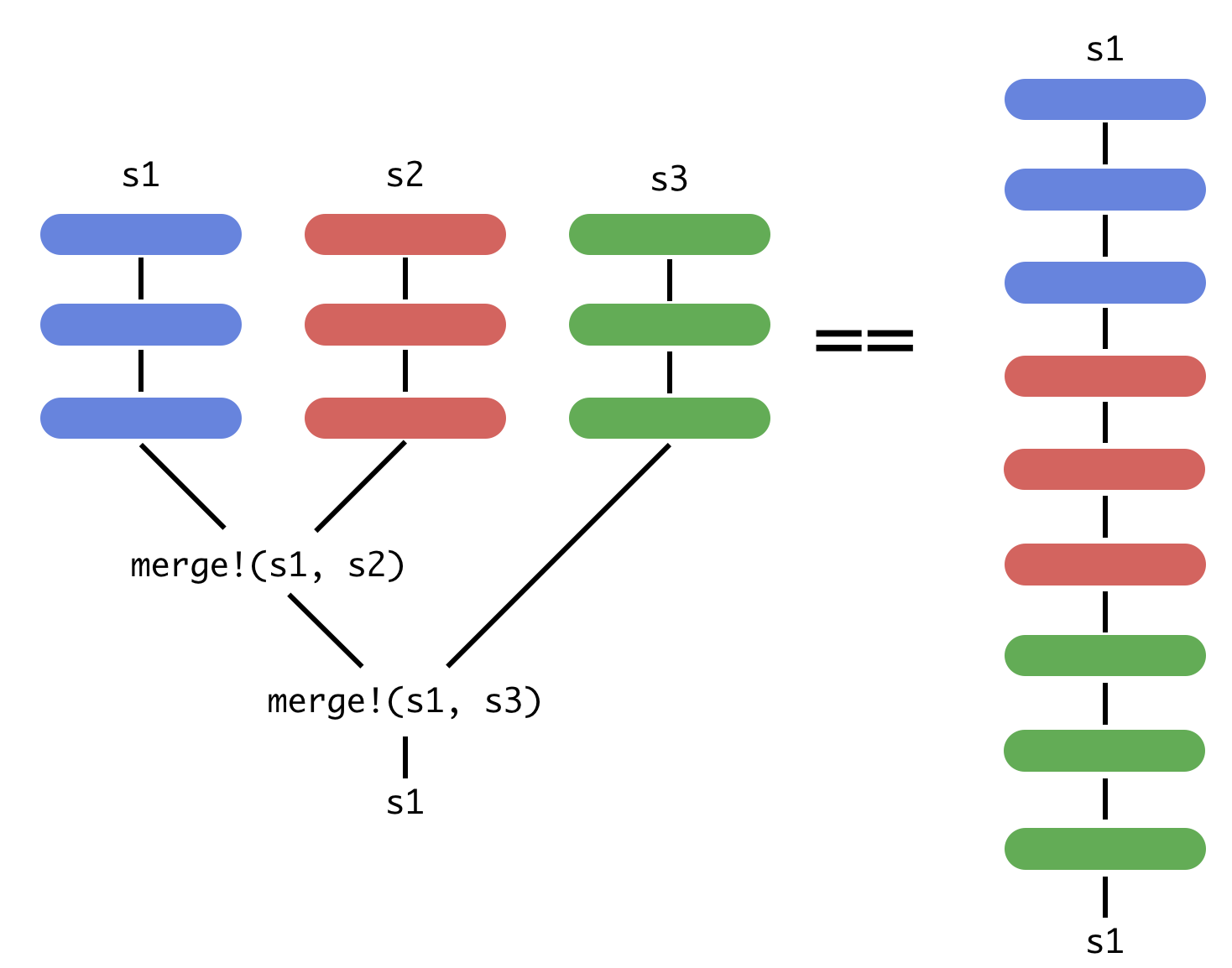• BLOG / NEWS

By Josh Day | Feb 27, 2019In many data science applications, it is easy to run out of memory when working with data. An analyst working with big data has a few options available to them:

2. Rent RAM with a cloud-based service.

3. Use a sample of the dataset.

None of these options are particularly good solutions.

With JuliaDB, one can easily read big data, save it in an efficient binary format, and even run operations out-of-core. Analytics are available via OnlineStats integration, making statistical calculations on big data a breeze.

OnlineStats implements on-line (single-pass) algorithms for statistics and models, meaning you can run analyses like linear regression on data that is too big to fit in memory. Every statistic/model in OnlineStats also supports merging, enabling parallel processing. The combination of on-line updating/merging eliminates the need for the entire dataset to be loaded into RAM simultaneously, allowing analyses that would not be possible with traditional methods. Below is a visualization of how JuliaDB integrates with OnlineStats by scheduling the updating and merging operations:# Example

From Kaggle's Huge Stock Market Dataset, there are over 7000 CSVs with historical price data (each stock's history in a different file). JuliaDB can quickly load them into a distributed dataset and perform group-by operations:

using Distributedaddprocs(4)@everywhere using JuliaDB, OnlineStats# 7195 CSVs with 14,887,665 rowsfiles = glob("*.txt", "Stocks")t = loadtable(files, filenamecol=:Stock)groupreduce(Mean(), t, :Stock; select=:Volume)
Distributed Table with 7163 rows in 4 chunks:Stock          Mean──────────────────────────────────────────────"a.us.txt"     Mean: n=4521 | value=3.9935e6"aa.us.txt"    Mean: n=12074 | value=3.33776e6"aaap.us.txt"  Mean: n=505 | value=1.49989e5"aaba.us.txt"  Mean: n=5434 | value=2.3218e7"aac.us.txt"   Mean: n=785 | value=2.20441e5"aal.us.txt"   Mean: n=989 | value=1.00454e7"aamc.us.txt"  Mean: n=1211 | value=17644.4"aame.us.txt"  Mean: n=2926 | value=6715.76"aan.us.txt"   Mean: n=3201 | value=7.13972e5"aaoi.us.txt"  Mean: n=1041 | value=7.94087e5"aaon.us.txt"  Mean: n=3201 | value=2.04258e5"aap.us.txt"   Mean: n=3201 | value=1.22519e6"aapl.us.txt"  Mean: n=8364 | value=1.06642e8"aat.us.txt"   Mean: n=1717 | value=2.15145e5"aau.us.txt"   Mean: n=3177 | value=1.86901e5"aav.us.txt"   Mean: n=3199 | value=4.28732e5"aaww.us.txt"  Mean: n=3162 | value=2.64112e5"aaxn.us.txt"  Mean: n=3201 | value=1.40883e6"ab.us.txt"    Mean: n=3201 | value=5.60349e5⋮

# Main Features

## Just-in-Time Compiled

JuliaDB leverages Julia’s just-in-time compiler (JIT) so that table operations – even custom ones – are fast.

## Compute in Parallel

Process data in parallel or even calculate statistical models out-of-core through integration with OnlineStats.jl.

## Store Any Data Type

JuliaDB supports Strings, Dates, Float64… and any other Julia data type, whether built-in or defined by you.

## Fast User-Defined Functions

JuliaDB is written 100% in Julia. That means user-defined functions are JIT compiled.

## Fast CSV Parser

CSVs are loaded extremely fast! Many files can be read at the same time to create a single table.

## Open Source

Distributed ComputingData larger than memoryMultiple IndexesCompiled UDFs